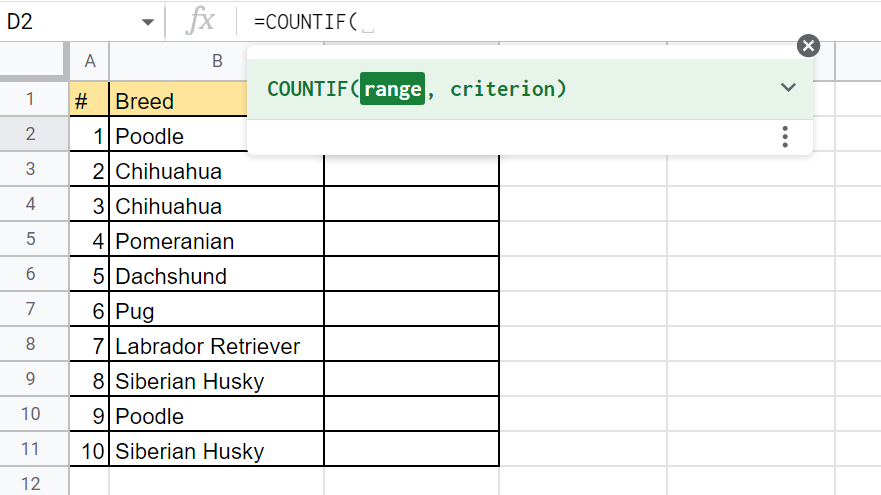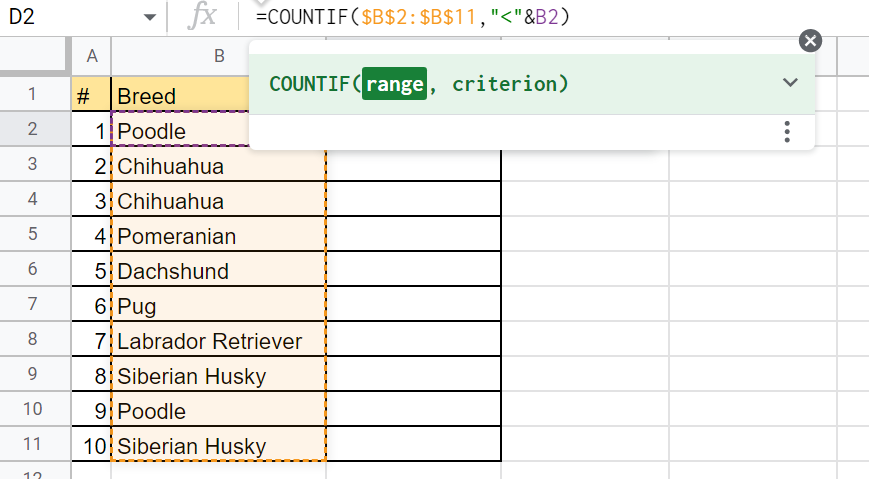# How to Rank Text Uniquely in Google Sheets

This guide will explain how you can use the COUNTIF function to rank text uniquely in Google Sheets.

With the COUNTIF function, we can determine how many unique values are higher than the current value.

When ranking text, you have a few ways to handle duplicate values. For example, if there is a tie for first place, you can give both entries a rank of 1. The next highest value will then get a rank of 3.

This type of ranking is common in competitions. If two people tie for first, both are recognized for 1st place, and the next competitor will receive third place.

However, you may sometimes want to rank text uniquely. In case of duplicates, you can give the higher rank to whoever appears first in the dataset. With this type of ranking, we do not skip over any number.

Let’s take a look at a quick example where we can rank text uniquely in Google Sheets.

You want to rank a series of text strings in alphabetical order. For example, given a list of months, April will rank first, August will rank second, and so on.

If there are duplicates in the list, you want to prioritize whichever string comes first. This ensures that given a list of size N, each string is assigned a ranking from 1 to N.

To perform this in Google Sheets, we can use the `COUNTIF` function. This function counts how many cells in a range follow a certain criteria. In this particular use case, we want to count how many other strings come before the current string to rank.

Now that we know when to use the `COUNTIF` function, let’s look at some sample tables in Google Sheets that use it to rank text.

## A Real Example of Ranking Text Uniquely in Google Sheets.

Let’s take a look at a real example of the `COUNTIF` function being used in a Google Sheets spreadsheet to rank text uniquely

In the example below, we have a table with 10 strings that indicate a specific dog breed. In columns C and D, we could rank the text according to their alphabetical order. Column C uses the normal ranking where equal strings receive the same ranking. For example, the string ‘Chihuahua’ appears twice but are both ranked first.The ranking in column D gives each string a unique ranking. In cases where the strings are equal, this ranking formula ranks whichever comes first higher.

When we get the values in Column C, we used the following formula:

`=COUNTIF(\$B\$2:\$B,"<"&B2) + 1`

To rank text normally, we simply count the number of values that come before the current string and add 1.

To give each string a unique ranking, we’ll have to modify this formula slightly:

`=COUNTIF(\$B\$2:\$B,"<"&B2) + COUNTIF(\$B\$2:B2,B2)`

In the formula above, instead of adding 1, we use `COUNTIF` again to check how many times the current string has appeared in the table. If the current string is the first instance, then this `COUNTIF` will return 1. However, in succeeding occurrences, this `COUNTIF` will continue incrementing the ranking.

In the example below, we can observe how the unique ranking differs from a normal ranking. If the text is ordered alphabetically, the unique rank should be its position in the list. Meanwhile, the normal ranking method skips certain numbers when there are duplicates.You can make your own copy of the spreadsheet above using the link attached below.

If you’re ready to try out this method of ranking text uniquely in Google Sheets, let’s start writing it ourselves!

## How to Rank Text Uniquely in Google Sheets

This section will guide you through each step needed to start using the `COUNTIF` function in Google Sheets. You’ll learn how we can use the `COUNTIF` function to determine the unique rank of a particular text string in a range.

Follow these steps to start using the `COUNTIF` function:

1. First, select the cell that will compute the rank. In this example, we’ll start with cell D2.2. Next, type ‘=COUNTIF(‘ in the Formula bar to start our `COUNTIF` function.3. The first argument of `COUNTIF` determines what range to check for values that fit a certain criteria. In this example, we’ll select the range B2:B11. We’ll convert it to an absolute reference by adding the ‘\$’ symbol.4. Next, we’ll type in the criterion for `COUNTIF`’s second argument. Since we want to determine how many cells in a range come before the current cell, we’ll concatenate the less than operator ‘<’ and a reference to the current cell.5. We’ll add the result of this function with another `COUNTIF` instance. In the second `COUNTIF`, we want our first argument to be the list of strings up to the current string. For the criterion, we will indicate a cell reference to the current cell. Hit the Enter key to return the final unique ranking.6. To determine the ranking of the remaining text values, simply use the Fill Handle tool and drag the first formula down until the entire column is filled.## Frequently Asked Questions (FAQ)

1. Can we rank text in reverse alphabetical order?
Yes, we can rank text in reverse alphabetical order by subtracting the unique ranking from the total number of values and adding one. For example, if we have a value that is ranked 7th place out of 10 values, then we can compute the reverse ranking through the formula =10-7+1 = 4.

This step-by-step guide should be all you need to start ranking text uniquely in Google Sheets. Our guide shows how we can use the `COUNTIF` function to count text strings based on their alphabetical order.

The `COUNTIF` function is just one of many Google Sheets functions you can use to analyze your dataset. With so many other Google Sheets functions available, you can surely find one that suits your use case.

Are you interested in learning more about what Google Sheets can do? Do subscribe to our Google Sheets newsletter to keep up with the latest guides and tutorials from us.### Get emails from us about Google Sheets.

Our goal this year is to create lots of rich, bite-sized tutorials for Google Sheets users like you. If you liked this one, you'd love what we are working on! Readers receive ✨ early access ✨ to new content.

##### You May Also Like## How To Use NOW Function In Google Sheets

The NOW function in Google Sheets is useful if you want to return the current date and time…## How to Use COLUMN Function in Google Sheets

The COLUMN function in Google Sheets is useful to get the column number of a specific cell. As…## How to Share Specific Tabs in Google Sheets

Learning to share specific tabs in Google Sheets may come in useful when you want to share a…## How to Use IFERROR Function in Google Sheets

The IFERROR function in Google Sheets is used to effectively manage errors. Instead of showing an error message,…